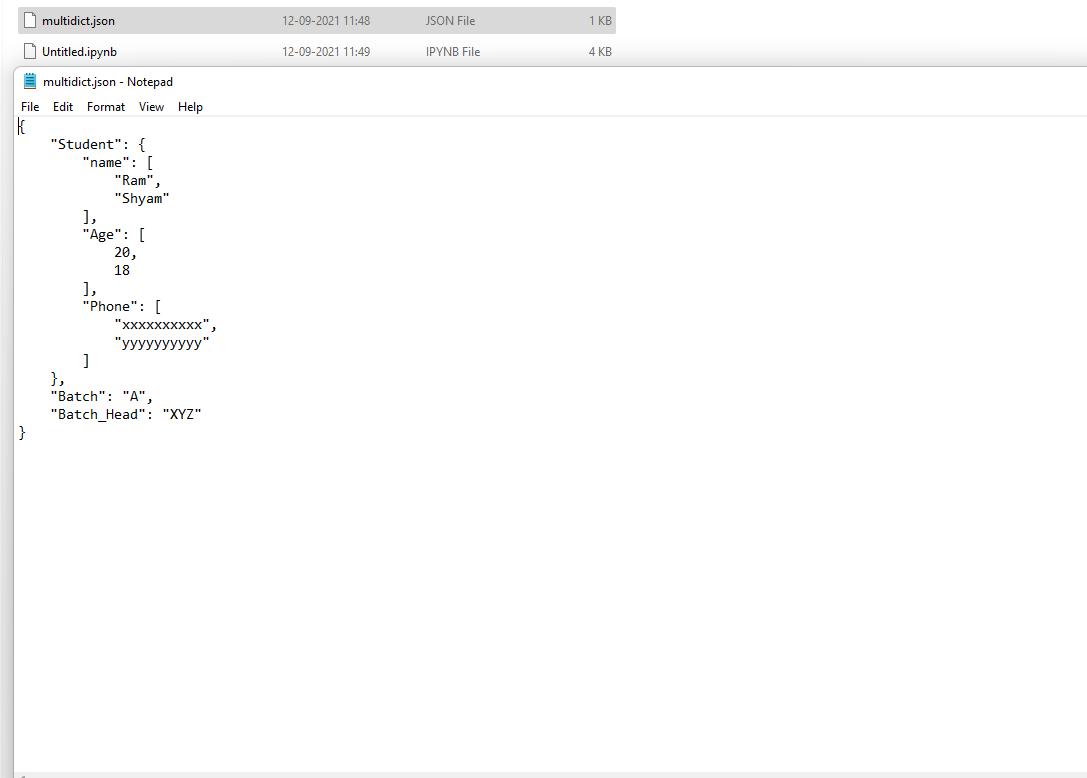# Converting MultiDict to proper JSON

• Last Updated : 02 Dec, 2021

In this article, we will see what is MultiDict, and how do we convert a multidict into JSON files in Python.

First, we will convert a multidict to a dictionary data type, and at last, we dump that dictionary into a JSON file.

Attention geek! Strengthen your foundations with the Python Programming Foundation Course and learn the basics.

To begin with, your interview preparations Enhance your Data Structures concepts with the Python DS Course. And to begin with your Machine Learning Journey, join the Machine Learning - Basic Level Course

## Functions Used :

• json.dump(): JSON module in Python module provides a method called dump() which converts the Python objects into appropriate JSON objects. It is a slight variant of the dumps() method.

Syntax : json.dump(d, skipkeys=False, ensure_ascii=True, check_circular=True, allow_nan=True, cls=None, indent=None, separators=None)

Parameters:

indent: It improves the readability of the JSON file. The possible values that can be passed to this parameter are simply double

quotes(“”), any integer values. Simple double quotes make every key-value pair appear in a new line.

Example :

json.dump(dic,file_name, indent=4)

• Converting MultiDict to proper JSONMultiDict: It is a class that is present in multidict python module

Syntax :

• multidict.MultiDict(**kwargs)
• multidict.MultiDict(mapping, **kwargs)
• multidict.MultiDict(iterable, **kwargs)

Return :

• It will create a mutable multidict instance.
• It has all the same functions that are available for dictionaries.

Example :

from multidict import MultiDict

dic = [(‘geeks’, 1), (‘for’,2), (‘nerds’, 3)]

multi_dict = MultiDict(dic)

Code:

## Python3

 `# import multidict module``from` `multidict ``import` `MultiDict``# import json module``import` `json` `# create multi dict``dic ``=` `[(``'Student.name'``, ``'Ram'``), (``'Student.Age'``, ``20``),``       ``(``'Student.Phone'``, ``'xxxxxxxxxx'``),``       ``(``'Student.name'``, ``'Shyam'``), (``'Student.Age'``,``18``),``       ``(``'Student.Phone'``, ``'yyyyyyyyyy'``),``       ``(``'Batch'``, ``'A'``), (``'Batch_Head'``, ``'XYZ'``)]` `multi_dict ``=` `MultiDict(dic)``print``(``type``(multi_dict))``print``(multi_dict)` `# get the required dictionary``req_dic ``=` `{}``for` `key, value ``in` `multi_dict.items():``  ` `      ``# checking for any nested dictionary``    ``l ``=` `key.split(``"."``)``    ` `    ``# if nested dictionary is present``    ``if` `len``(l) > ``1``: ``        ``i ``=` `l[``0``]``        ``j ``=` `l[``1``]``        ``if` `req_dic.get(i) ``is` `None``:``            ``req_dic[i] ``=` `{}``            ``req_dic[i][j] ``=` `[]``            ``req_dic[i][j].append(value)``        ``else``:``            ``if` `req_dic[i].get(j) ``is` `None``:``                ``req_dic[i][j] ``=` `[]``                ``req_dic[i][j].append(value)``            ``else``:``                ``req_dic[i][j].append(value)` `    ``else``:  ``# if single dictionary is there``        ``if` `req_dic.get(l[``0``]) ``is` `None``:``            ``req_dic[l[``0``]] ``=` `value``        ``else``:``            ``req_dic[l[``0``]] ``=` `value``# save the dict in json format``with ``open``(``'multidict.json'``, ``'w'``) as ``file``:``    ``json.dump(req_dic, ``file``, indent``=``4``)`

Output:

<class ‘multidict._multidict.MultiDict’>

<MultiDict(‘Student.name’: ‘Ram’, ‘Student.Age’: 20, ‘Student.Phone’: ‘xxxxxxxxxx’, ‘Student.name’: ‘Shyam’, ‘Student.Age’: 18, ‘Student.Phone’: ‘yyyyyyyyyy’, ‘Batch’: ‘A’, ‘Batch_Head’: ‘XYZ’)>

JSON file output:My Personal Notes arrow_drop_up